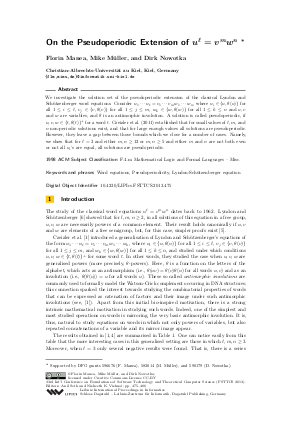Document# On the Pseudoperiodic Extension of u^l = v^m w^n

### Authors Florin Manea, Mike Müller, Dirk Nowotka## File

LIPIcs.FSTTCS.2013.475.pdf
• Filesize: 0.5 MB
• 12 pages

## Cite As

Florin Manea, Mike Müller, and Dirk Nowotka. On the Pseudoperiodic Extension of u^l = v^m w^n. In IARCS Annual Conference on Foundations of Software Technology and Theoretical Computer Science (FSTTCS 2013). Leibniz International Proceedings in Informatics (LIPIcs), Volume 24, pp. 475-486, Schloss Dagstuhl - Leibniz-Zentrum für Informatik (2013)
https://doi.org/10.4230/LIPIcs.FSTTCS.2013.475

## Abstract

We investigate the solution set of the pseudoperiodic extension of the classical Lyndon and Sch\"utzenberger word equations. Consider u_1 ... u_l = v_1 ... v_m w_1 ... w_n, where u_i is in {u, theta(u)} for all 1 <= i <= l, v_j is in {v, theta(v)} for all 1 <= j <= m, w_k is in {w, theta(w)} for all 1 <= k <= n and u, v and w are variables, and theta is an antimorphic involution. A solution is called pseudoperiodic, if u,v,w are in {t, theta(t)}^+ for a word t. [Czeizler et al./I&C/2011] established that for small values of l, m, and n non-periodic solutions exist, and that for large enough values all solutions are pseudoperiodic. However, they leave a gap between those bounds which we close for a number of cases. Namely, we show that for l = 3 and either m,n >= 12 or m,n >= 5 and either m and n are not both even or not all u_i's are equal, all solutions are pseudoperiodic.
##### Keywords
• Word equations
• Pseudoperiodicity
• Lyndon-Schützenberger equation

## Metrics

• Access Statistics
• Total Accesses (updated on a weekly basis)
0